# Weight Relations & Inter Relations Notes | Study Soil Mechanics - Civil Engineering (CE)

## Civil Engineering (CE): Weight Relations & Inter Relations Notes | Study Soil Mechanics - Civil Engineering (CE)

The document Weight Relations & Inter Relations Notes | Study Soil Mechanics - Civil Engineering (CE) is a part of the Civil Engineering (CE) Course Soil Mechanics.
All you need of Civil Engineering (CE) at this link: Civil Engineering (CE)

Weight Relations

Density is a measure of the quantity of mass in a unit volume of material. Unit weight is a measure of the weight of a unit volume of material. Both can be used interchangeably. The units of density are ton/m³, kg/m³ or g/cm³. The following are the basic weight relations:

1. The ratio of the mass of water present to the mass of solid particles is called the water content (w), or sometimes the moisture content.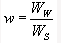Its value is 0% for dry soil and its magnitude can exceed 100%.

2. The mass of solid particles is usually expressed in terms of their particle unit weight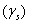or specific gravity (Gs) of the soil grain solids .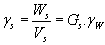where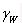= Unit weight of water

For most inorganic soils, the value of Gs lies between 2.60 and 2.80. The presence of organic material reduces the value of Gs.

3. Dry unit weight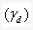is a measure of the amount of solid particles per unit volume.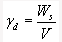4. Bulk unit weight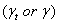is a measure of the amount of solid particles plus water per unit volume.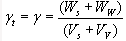5. Saturated unit weight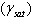is equal to the bulk density when the total voids is filled up with water.

6. Buoyant unit weight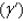or submerged unit weight is the effective mass per unit volume when the soil is submerged below standing water or below the ground water table.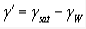Inter-Relations

It is important to quantify the state of a soil immediately after receiving in the laboratory and prior to commencing other tests. The water content and unit weight are particularly important, since they may change during transportation and storage.

Some physical state properties are calculated following the practical measurement of others. For example, dry unit weight can be determined from bulk unit weight and water content. The following are some inter-relations: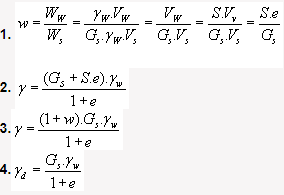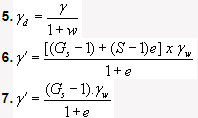The document Weight Relations & Inter Relations Notes | Study Soil Mechanics - Civil Engineering (CE) is a part of the Civil Engineering (CE) Course Soil Mechanics.
All you need of Civil Engineering (CE) at this link: Civil Engineering (CE)Use Code STAYHOME200 and get INR 200 additional OFF

## Soil Mechanics

31 videos|49 docs|42 tests

Track your progress, build streaks, highlight & save important lessons and more!

,

,

,

,

,

,

,

,

,

,

,

,

,

,

,

,

,

,

,

,

,

;